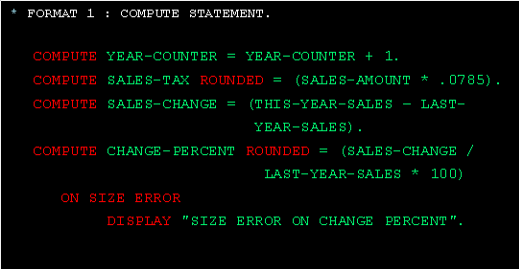## Thursday, 4 September 2014

### COBOL Compute Statement | COBOL DIVIDE Verb Example.COBOL Compute Statement.
The Compute statement calculates the arithmetic expression to the right of the equals sign and stores the result in the variable to the left of the equals sign.
Within the expression, you use the arithmetic operators for addition, subtraction, multiplication (*), division, and exponentiation (**). Exponentiation means “raise to the power of” so A ** 2 is the same as A2.
All variables in the arithmetic expression must be numeric items. The variable that will contain the result of the arithmetic expression can be a numeric edited item if that variable isn’t used in the arithmetic expression. Otherwise, it must be numeric.
You can code the Rounded clause whenever the result of a calculation can have more decimal places than are specified in the picture of the result field. If you don’t use the Rounded clause, the extra decimal places are truncated.
You can code the On Size Error clause when there’s a chance that the result may be larger than the receiving field. If it is, the statements in this clause are executed.
Format :
COMPUTE data-name [ROUNDED] = arithmetic-expression
[ON SIZE ERROR statement-group]

Example:COBOL Compute Statement.